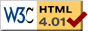### Journée-séminaire de combinatoire

#### (équipe CALIN du LIPN, université Paris-Nord, Villetaneuse)

Le 25 février 2015 à 14h00 en Amphi A, Michael Drmota nous parlera de : Quasi-random properties of subsequences of sequences generated by finite automata

Résumé : Automatic sequences T(n) are the output sequence of a finite automaton, where the input is the q-adic digital representation of n. The most prominent example is the Thue-Morse sequence t(n) (that is also the fixed point of the substitution 0 -> 01, 1 -> 10). Automatic sequences have been studied in many diff erent contexts from combinatorics to algebra, number theory, harmonic analysis, geometry and dynamical systems. For example, they have a linear subword complexity and they are almost periodic. Since the subword complexity is linear the entropy of the related dynamical system is zero. This also means that they do not behave like a random sequence. However, the situation changes drastically when one uses proper subsequences of automatic sequences, for example the subsequence along primes or squares. It is conjectured that the resulting sequences are normal sequences so that they behave like random sequences (=quasi random sequences). Recently this property was proved (together with C. Mauduit and J. Rivat) for the Thue-Morse sequence along the subsequence of squares - and it turns out that this propery extends to several other automatic sequences and also to subsequences of the form [n^c], where 1 < c < 4/3. Thus such subsequences of automatic sequences give rise to a completely new class of pseudo random sequences that can be computed very efficiently.

 Dernière modification : Monday 24 January 2022Contact pour cette page : Cyril.Banderier at lipn.univ-paris13.fr